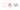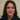# Comparing Array.from and spread syntax in Javascript with examples

Definitively Array.from and spread syntax in Javascript are different from each other. Although we could use them in similar situations.

## Array.from and spread syntax definintion

### Array.from

According to MDN with `Array.from()` method we can create a new, shallow-copied `Array` instance from an array-like or iterable object. So it means you can create arrays from:

### Spread syntax

Spread syntax allows an iterable to expand in places where 0+ arguments are expected. It does not work on array-like objects (i.e. objects that have the `length` property and indexed elements) .

## Copying arrays

As an example we can copy arrays with both methods just like this:

``````// Using Array.from
let a1 = [1,2,3];
let a2 = Array.from(a1);
a2.push(4)
a2 = [1, 2, 3, 4]``````
``````// Using spread syntax
let a1 = [1,2,3];
let a2 =[ ...a1];
a2.push(4)
a2 = [1, 2, 3, 4]``````

As a result we can use both in these case but the spread syntax is shorter and more readable.

## Create array from a map and set

Eventually we can create arrays from mapped objects or a set object using both array.from and spread syntax in practice:

``````var m = new Map([[1, 2], [2, 4], [4, 8]]);<br/><br/>// Using from method
Array.from(m);

// Using spread syntax
[...m];``````

Moreover we can use them both on a set object:

``````var s = new Set(['foo', window]); <br/><br/>//Using from method<br/>Array.from(s);<br/><br/>//Using spread syntax
[...s];``````

As a result in this case as well spread syntax is a better option as it makes the code look better.

### map functions inside Array.from method

Comparably we better know `Array.from()` has an optional parameter `mapFn`, which allows us to execute a `map` function on each element of the array (or subclass object) that is being created. Therefor we can use map function inside it like below:

``````// Using an arrow function as the map function to
// manipulate the elements
Array.from([1, 2, 3], x => x + x);
// [2, 4, 6]``````

### Array-like objects

However we only can use Array.from if we wanted to work on array-like objects. Take a look at this example:

``````function f() {
return Array.from(arguments);
}

const result = f(1, 2, 3);

// result =  [1, 2, 3]``````

### Destructuring with spread

Practically one of the best funs using spread is with destructuring. Have a look at this example:

``````let { x, y, ...z } = { x: 1, y: 2, a: 3, b: 4 };
console.log(x); // 1
console.log(y); // 2
console.log(z); // { a: 3, b: 4 }``````

Moreover here is a thorough article on this topic. Thankfully we can use spread syntax in most use cases and we better do. I looked at just a few usages here. It is very short and more maintainable and readable. Although there are some use cases which we have to use Array.from as mentioned. Thanks for reading.

© 2020This site is created and maintined by Azadeh Faramarzi , A passionate developer, sport and code lover who loves to share and write when she has some time.